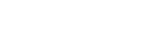# pourRNA

Compute local minima and respective transition rates of an RNA energy landscape.

The source code can be obtained from the GitHub page.

To install this package with conda run:

``conda install -c bioconda pourrna``

# Execute

The minimal input is an RNA sequence. Either per standard input (from a *.fasta file)

``````cat rna.fasta | pourRNA
``````

or per command line argument

``````pourRNA --sequence="CUAGUUAGGAACGGAAUUAAUUAGGAAAAAGCUGAUUAG"
``````

The content of the file rna.fasta should look similar to this:

``````> fasta header
CUAGUUAGGAACGGAAUUAAUUAGGAAAAAGCUGAUUAG
``````

The output consists of the representative structures of the local minima and the transition rates between adjacent local minima. You can adjust the output and speed up the computation by using additional command line parameters. All parameters are shown by

``````pourRNA --help
``````

# Post-processing

If you are only interested in the thermodynamic equilibrium of the markov process, you can simply extract the line with the equilibrium densities for the local minima:

``````cat rna.fasta | pourRNA | grep "Equilibrium Densities:" -A1
``````

If you are interested in the dynamic folding behaviour, you need additional tools in order to post-process the output of pourRNA. Usually the tool treekin is used, which needs two input files that should have the output format of the tool 'barriers'. pourRNA can also be used to produce a barriers-like output.

``````cat rna.fasta | pourRNA --barriers-like-output=rna_barriers
``````

As a second step you need one or several start structures for the initial population of the markov process. In this example we extract the open chain structure from the barriers like output:

``````cat rna_barriers_states.out | grep -P "\s*\d+\s[\.]+\s+\-?\d*\.?\d*"
``````

In this example the index of our start structure is 34. With this we can start treekin:

``````cat ./rna_barriers_rates.out | treekin -m I --bar=./rna_barriers_states.out --p0 34=1.0 > treekin.out
``````

The treekin output is a matrix with the time steps in the first column and the population densities for all local minima in the other columns. This file can be visualized for example with the tool 'gracebat'.

``````gracebat -log x -nxy treekin.out -hdevice PostScript -hardcopy -printfile kinetics.ps
ps2pdf kinetics.ps
``````

The final kinetics.pdf shows the folding process from the initial population until the thermodynamic equilibrium is reached.

If you don't have access to treekin and want to use the R script within the scripts directory of this project, you can compute the kinetics pdf file as follows:

``````cat rna.fasta | pourRNA --barriers-like-output=rna_barriers --binary-rates-file=rna_rate_matrix
Rscript ./scripts/read_matrix_plot_kinetics.R --binary_matrix ./rna_rate_matrix --states_file ./rna_barriers_states.out --initial_state=34``````

The output of this call is the file rna_rate_matrix.pdf within the current directory.

The kinetics image should look similar to this: